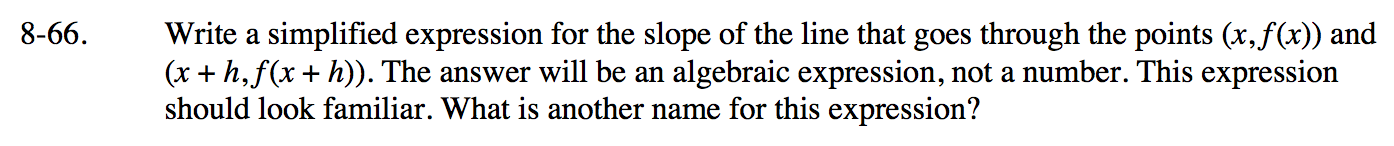### Home > PC3 > Chapter 8 > Lesson 8.2.1 > Problem8-66

8-66.

Write a simplified expression for the slope of the line that goes through the points (x, f (x)) and (x + h, f (x + h)). The answer will be an algebraic expression, not a number. This expression should look familiar. What is another name for this expression? Homework Help ✎$\frac{f(x+h)-f(x)}{(x+h)-x}$